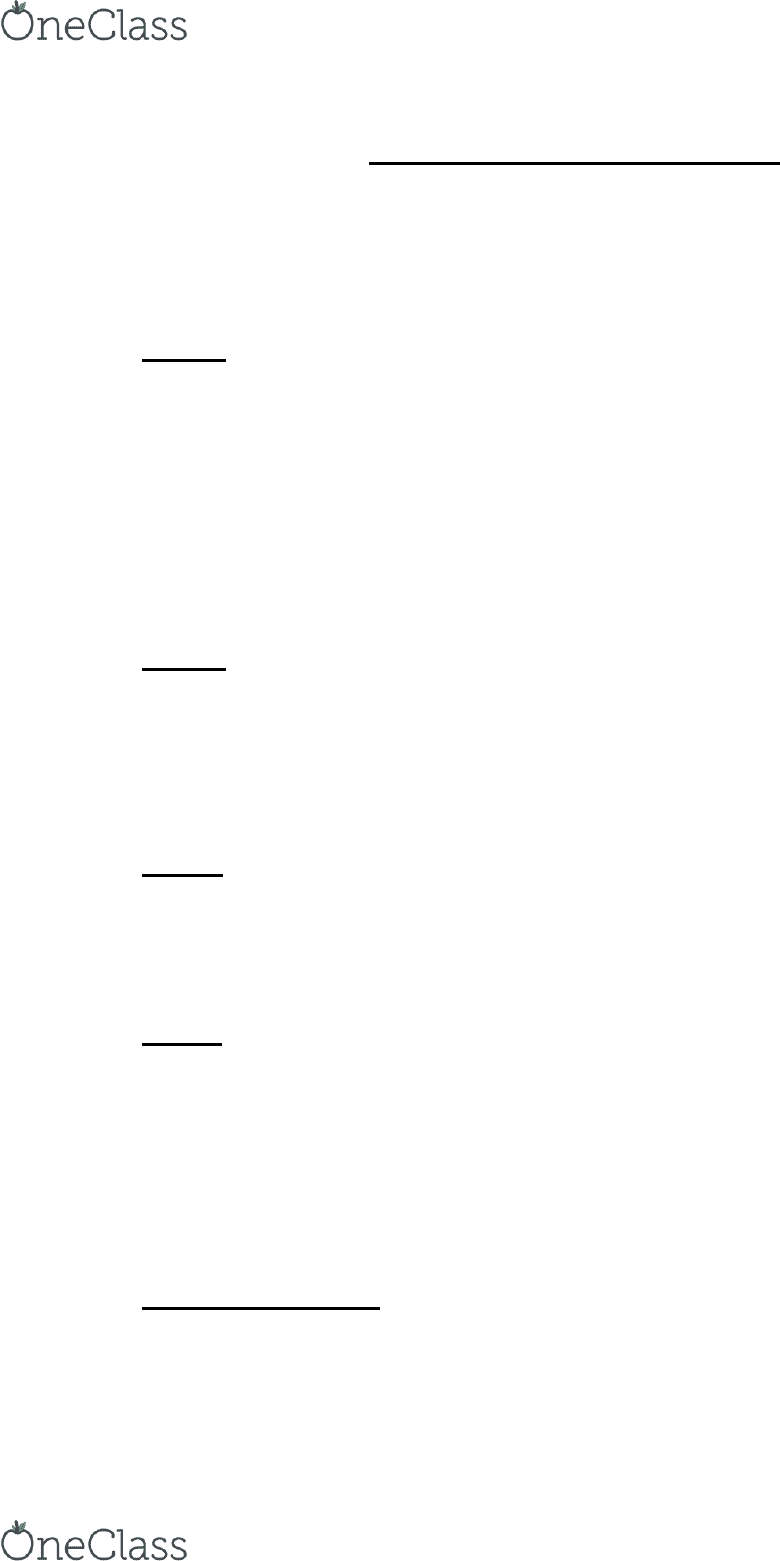Textbook Notes (280,000)
CA (170,000)
UOttawa (6,000)
PHY (20)
Chapter 1

# PHY1331 Chapter 1: PHY1331 – Chapter 1 Textbook Notes

Department
Physics
Course Code
PHY 1331
Professor
Selahattin Celebi
Chapter
1

This preview shows half of the first page. to view the full 3 pages of the document.PHY1331 – Chapter 1 Textbook Notes
Classical mechanics (Newtonian)  “motion of objects that are large relative to
atoms and move at speeds much slower than the speed of light”
Mechanics include length, mass and time, but must choose a standard.
SI Units
Length  meter (m)
Mass  kilogram (kg)
Time  second (s)
Temperature  kelvin
Electric current  ampere
Amount of substance  mole
Fundamental Quantities are: length, time, mass
Derived Quantities are: area, speed, density
Density
Symbol is p
Measured in kg/m3
Equation p = m/V
oV = m/p
om = pV
Review
- Proton carries a positive charge
- Atomic number identifies the number of protons of a chemical element
- Mass number is the number of protons and neutrons (can vary)
- [ ] = means dimensions
Quarks
- 6 different particles that include both protons and neutrons
oup = +2/3
odown = -1/3
ostrange = -1/3
ocharmed = +2/3
obottom = -1/3
otop = +2/3
Dimensional Analysis
- a method used to simplify problems
- quantities only be added/subtracted if they have the same dimensions
- Area (A) = L2 (m2)
- Volume (V) = L3 (m3)
find more resources at oneclass.com
find more resources at oneclass.com